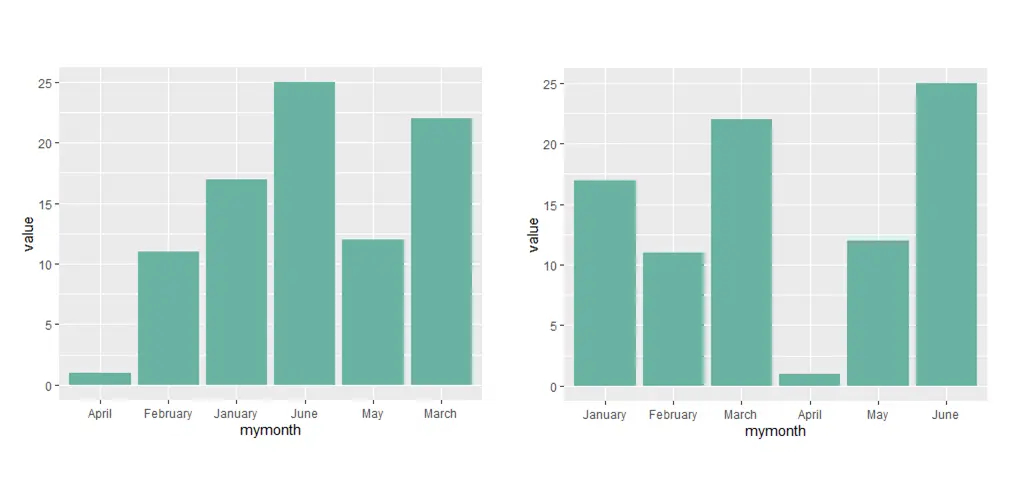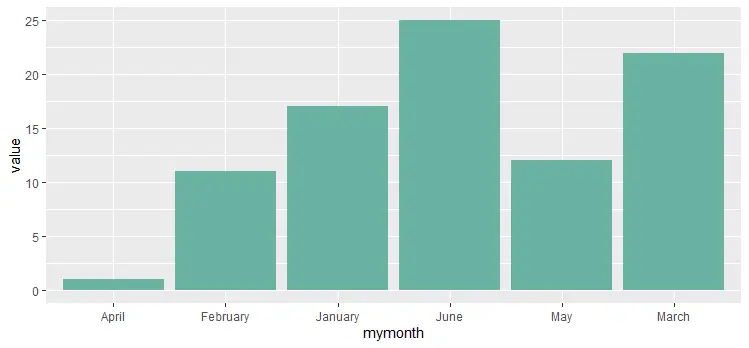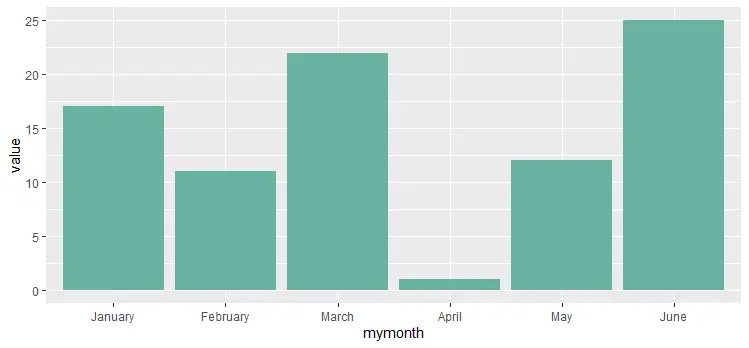# Sort text as numbers in R

Sometimes it might be useful to sort text as numbers in R. For example, if you have a month’s names and it is necessary to show them in chronological order in ggplot. Even if the content is already in the right order, it doesn’t work that way on the x-axis.

Here is my data frame that contains month names and values. As you can see, the month names are in the right order.

```df <- data.frame(
mymonth = c("January", "February", "March", "April", "May", "June") ,
value = c(17, 11, 22, 1, 12, 25)
)

df

#   mymonth value
#1  January    17
#2 February    11
#3    March    22
#4    April     1
#5      May    12
#6     June    25```

After creating a bar plot with the ggplot2 package, month names are in alphabetical order.

```require(ggplot2)

ggplot(df, aes(x = mymonth, y = value)) +
geom_bar(stat = "identity", fill = "#69b3a2")```To reorder the ggplot x-axis and categories in the necessary order, you should sort text as numbers in R.

To add these capabilities to text, use the factor function to replace the month vector with encoded as a factor. In this case, months are in the right order, and transformation goes with a unique list of them. If that is not your case, take a look below.

`df\$mymonth <- factor(df\$mymonth, levels = unique(df\$mymonth))`

In the result, the month column is a factor.

```class(df\$mymonth)

# "factor"```

The plot can use that to show the x-axis in the necessary order.

```ggplot(df, aes(x = mymonth, y = value)) +
geom_bar(stat = "identity", fill= "#69b3a2")```Here is another example. Data frames have month names, but they are not in the right order.

```df <- data.frame(
mymonth = c("June", "May", "April", "March", "February", "January") ,
value = c(25, 12, 1, 22, 11, 17)
)

df

#   mymonth value
#1     June    25
#2      May    12
#3    April     1
#4    March    22
#5 February    11
#6  January    17```

If you try to sort this data frame by month column, it will do that alphabetically.

```df[order(df\$mymonth), ]

#   mymonth value
#3    April     1
#5 February    11
#6  January    17
#1     June    25
#2      May    12
#4    March    22
```

In that case, I’m using a vector that defines numeric order.

```df\$mymonth <-
factor(df\$mymonth,
levels = c("January", "February", "March", "April", "May", "June"))```

As a result, I can sort text values as numbers in R.

```df[order(df\$mymonth), ]

#   mymonth value
#6  January    17
#5 February    11
#4    March    22
#3    April     1
#2      May    12
#1     June    25```

Please take a look at other posts in this blog. For example, posts that contain data visualization.

If you like tips and tricks in R, I recommend you a collection that involves dplyr or RStudio.

Categories R